# The freezer

1. The temperature inside a freezer is minus 23 degree Celsius. The temperature falls by a further 12 degree degree Celsius. What is the new temperature?
2. What is the difference between temperatures of 12 degree Celsius and 210 degree Celsius?

Correct result:

a =  -35 °C
b =  -198 °C

#### Solution:

$a=-23-12=-3{5}^{\circ }\text{C}$
$b=12-210=-19{8}^{\circ }\text{C}$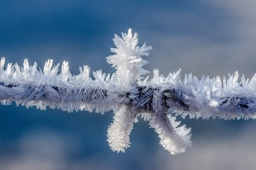We would be pleased if you find an error in the word problem, spelling mistakes, or inaccuracies and send it to us. Thank you!## Next similar math problems:

• MW oven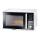You plug a microwave oven into a 20 amp electrical circuit. the microwave uses as much as 12.5 amps . How many amps are available on this circuit for other appliances?
• KingKing had four sons. First inherit 1/2, second 1/4 , third 1/5 of property. What part of the property was left to the last of the brothers?
• Celsius degreesThe temperature on Monday was 5 celsius. The temperature on Thursday was 7 degrees less than the temperature on Monday. What was the temperature on Thursday?
• Midnight 2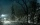It was 8 degrees at nightfall. The temperature dropped 10 by midnight. What was the temperature at midnight?
• The temperature 3The temperature is -12 degrees Fahrenheit. During the following 5 hours the temperature decrease 23 degrees Fahrenheit what is the temperature at 11 pm?
• The temperature 2The temperature of Siberia fell13 degrees below 2/3 degrees centigrad. What is the temperature there now?
• The outdoorThe outdoor temperature was 60 degrees Fahrenheit at midnight. The temperature went down 3 degrees each hour for the next 4 hours. What was the temperature at 4 A. M. ?
• Storm 3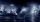If the temperature yesterday was 56 and today is 13 degrees cooler, what is today's temperature?
• Celsius 25 degrees Celsius at midday dropped 12°Celsius degree by evening. What is the temperature?
• Difference of temperaturesThe temperature on Saturday was –2°C. The temperature on Sunday was 8°C. Write down the difference in these two temperatures.
• 8 degrees8 degrees Celsius it cools down by 9 degrees Celsius. What is the resultant temperature?
• Melting point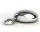The melting point of mercury is -36°F and its boiling point is 672°F. What is the difference between the boiling point and the melting point?
• Degrees 2The temperature was 3°F and falls four degrees Fahrenheit. What is actually temperature?
• The lowestThe lowest temperature on Thursday was 20°C. The lowest temperature on Saturday was 12°C. What was the difference between the lowest temperatures?
• Temperature differenceThe highest temperature of Libya was recorded 58 degree Celsius and the lowest was recorded -88 degree Celsius, what is the temperature difference?
• On SundayOn Sunday the temperature reached a high of 38° above 0. That night it dropped to 23° below 0. What is the difference between the high and low temperatures for Sunday?
• Outdoor temperatureThe outdoor temperature was 8 degrees at midnight. The temperature declined 5 degrees during each of the next 3 hours. What was the temperature at 3am?